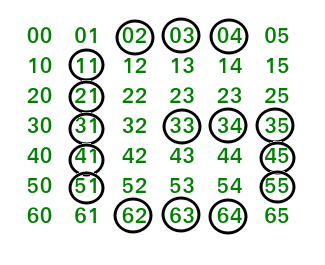Related Articles

# Program to print the pattern ‘G’

• Difficulty Level : Hard
• Last Updated : 07 Apr, 2021

In this article, we will learn how to print the pattern G using stars and white-spaces. Given a number n, we will write a program to print the pattern G over n lines or rows.
Examples:

```Input : 7
Output :
***
*
*
* ***
*   *
*   *
***

Input : 9
Output :
*****
*
*
*
*   ***
*     *
*     *
*     *
*****  ```

Attention reader! All those who say programming isn't for kids, just haven't met the right mentors yet. Join the  Demo Class for First Step to Coding Coursespecifically designed for students of class 8 to 12.

The students will get to learn more about the world of programming in these free classes which will definitely help them in making a wise career choice in the future.

In this program, we have used the simple logic of iteration over lines to create the pattern G. Please look at the image below which represents the pattern G in the form of a 2-d matrix, where mat[i][j] = ‘ij’:If we try to analyze this picture with a (row, column) matrix and the circles represent the position of stars in the pattern G, we will learn the steps. Here we are performing the operations column-wise. So for the first line of stars, we set the first if condition, where the row position with 0 and (n-1) won’t get the stars and all other rows from 1 to (n-1), will get the stars. Similarly, for the second, third and fourth column we want stars at the position row = 0 and row = (n-1). The other steps are self-explanatory and can be understood from the position of rows and columns in the diagram.
Below is the implementation of above idea:

## C++

 `// C++ program to print the pattern G``#include ``using` `namespace` `std;` `void` `pattern(``int` `line)``{``    ``int` `i, j;``    ``for``(i = 0; i < line; i++)``    ``{``        ``for``(j = 0; j < line; j++)``        ``{``            ``if``((j == 1 && i != 0 && i != line - 1) ||``              ``((i == 0 || i == line - 1) && j > 1 &&``              ``j < line - 2) || (i == ((line - 1) / 2) &&``              ``j > 2 && j < line - 1) || (j == line - 2 &&``              ``i != 0 && i >= ((line - 1) / 2) && i != line - 1))``                ``printf``(``"*"``);``            ``else``                ``printf``( ``" "``);` `        ``}``        ``printf``(``"\n"``);``    ``}``}` `// Driver code``int` `main()``{``    ``int` `line = 7;``    ``pattern(line);``    ``return` `0;``}` `// This code is contributed``// by  vt_m.`

## Java

 `// Java program to print the pattern G``import` `java.io.*;` `class` `GFG {` `    ``static` `void` `pattern(``int` `line)``    ``{``        ``int` `i, j;``        ``for``(i = ``0``; i < line; i++)``        ``{``            ``for``(j = ``0``; j < line; j++)``            ``{``                ``if``((j == ``1` `&& i != ``0` `&& i != line - ``1``) ||``                ``((i == ``0` `|| i == line - ``1``) && j > ``1` `&&``                ``j < line - ``2``) || (i == ((line - ``1``) / ``2``) &&``                ``j > ``2` `&& j < line - ``1``) || (j == line - ``2` `&&``                ``i != ``0` `&& i >= ((line - ``1``) / ``2``) && i != line - ``1``))``                    ``System.out.print(``"*"``);``                ``else``                    ``System.out.print( ``" "``);``    ` `            ``}``            ``System.out.println();``        ``}``    ``}``    ` `    ``// Driver code``    ``public` `static` `void` `main (String[] args)``    ``{``        ``int` `line = ``7``;``        ``pattern(line);``    ``}``}` `// This code is contributed by vt_m.`

## Python

 `# Python program to print pattern G``def` `Pattern(line):``    ``pat``=``""``    ``for` `i ``in` `range``(``0``,line):   ``        ``for` `j ``in` `range``(``0``,line):    ``            ``if` `((j ``=``=` `1` `and` `i !``=` `0` `and` `i !``=` `line``-``1``) ``or` `((i ``=``=` `0` `or``                ``i ``=``=` `line``-``1``) ``and` `j > ``1` `and` `j < line``-``2``) ``or` `(i ``=``=` `((line``-``1``)``/``2``)``                ``and` `j > line``-``5` `and` `j < line``-``1``) ``or` `(j ``=``=` `line``-``2` `and``                ``i !``=` `0` `and` `i !``=` `line``-``1` `and` `i >``=``((line``-``1``)``/``2``))): ``                ``pat``=``pat``+``"*"`  `            ``else``:     ``                ``pat``=``pat``+``" "`  `        ``pat``=``pat``+``"\n"`  `    ``return` `pat`` ` `# Driver Code``line ``=` `7``print``(Pattern(line))`

## C#

 `// C# program to print the pattern G``using` `System;` `class` `GFG {` `    ``static` `void` `pattern(``int` `line)``    ``{``        ``int` `i, j;``        ``for``(i = 0; i < line; i++)``        ``{``            ``for``(j = 0; j < line; j++)``            ``{``                ``if``((j == 1 && i != 0 && i != line - 1) ||``                ``((i == 0 || i == line - 1) && j > 1 &&``                ``j < line - 2) || (i == ((line - 1) / 2) &&``                ``j > 2 && j < line - 1) || (j == line - 2 &&``                ``i != 0 && i >= ((line - 1) / 2) && i != line - 1))``                    ``Console.Write(``"*"``);``                ``else``                    ``Console.Write( ``" "``);``    ` `            ``}``            ``Console.WriteLine();``        ``}``    ``}``    ` `    ``// Driver code``    ``public` `static` `void` `Main ()``    ``{``        ``int` `line = 7;``        ``pattern(line);``    ``}``}` `// This code is contributed by vt_m.`

## PHP

 ` 1 ``and` `\$j` `< ``\$line``-2) ``or` `(``\$i` `== ((``\$line``-1)/2)``            ``and` `\$j` `> 2 ``and` `\$j` `< ``\$line``-1) ``or` `(``\$j` `== ``\$line``-2 ``and``            ``\$i` `!= 0 ``and` `\$i` `>=((``\$line``-1)/2) ``and` `\$i` `!= ``\$line``-1))``                ``echo` `"*"``;   ``            ``else``                ``echo` `" "``;    ``        ``}       ``      ``echo` `"\n"``;``    ``}``}` `// Driver Code``\$line` `= 7;``Pattern(``\$line``)``?>`

## Javascript

 ``

Output:

```  ***
*
*
* ***
*   *
*   *
***  ```

My Personal Notes arrow_drop_up